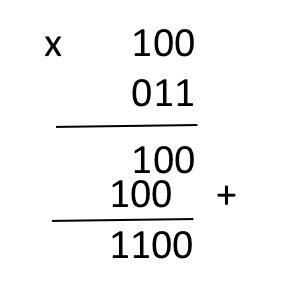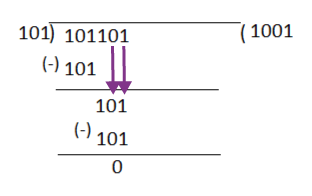# Binary Subtraction, Addition, Multiplication & Division

As we know Binary numbers consist of only 2 digits (bits) i.e. 0 & 1. Here, we will learn how to perform the binary subtraction, addition, multiplication, or division between any two binary bits.

```Example: 10001 + 11101
Solution:
1
1 0 0 0 1
+ 1 1  1  0 1
———————–
1 0 1 1 1 0```

## Binary Subtraction

```Example : 0011010 – 001100
Solution:
1 1 Borrow
0 0 1 1 0 1 0
(-) 0 0 1 1 0 0
——————
0 0 0 1 1 1 0```

## Binary Multiplication

```Example: 100 X 011```

## Binary Division

```Example: 101101 / 101```

## 1’s & 2’s complement methods of Binary Subtraction

Binary subtraction can be performed using One’s & Two’s complement methods. 1’s complement is another obtained by toggling (changing) all of its bits, i.e. converting the 0 bit to 1 and the 1 bit to 0. Whereas, 2’s complement is 1 plus the binary number’s 1’s complement.

For Example: 1’s complement of binary number 10101110 is 01010001. & 2’s complement of binary number 10101110 is 01010001 + 1 = 01010010

### 1’s complement of Binary Subtraction

Steps involved in 1’s complement of Binary Subtraction

1. Make both bits of Subtrehend & Minuend equal. (Here, Minuend is upper bit & Subtrahend is lower one)
2. Find the 1’s complement of Subtrehend.
3. Now, add 1’s complement of Subtrehend and Minuend.
4. Check the Overflow bit.
• If there is an overflow bit (carry-bit), add the carry-bit to the remaining portion.
• Else take 1’s complement of the result again which will be negative.

### Example 1 – Calculate 10101 – 00111 using 1’s complement

``` Step 1 - Both minuend & subtrahend are equal.
Step 2 - We take 1's complement of subtrahend 00111, which is 11000.
Step 3 - Now, sum them. So, 10101+11000 =1 01101.
Step 4 - In the above result, we get the carry bit 1. So the result must be positive. Here, we discard it and add it to the remaining sum i.e. 01101+1 = 01110 which is the final result.```

### Example 2 –  Calculate 101 – 1000 using 1’s complement.

``` Step 1 - Making minuend & subtrahend of equal bit we get, 0101 - 1000.
Step 2 - We take 1's complement of subtrahend 1000, which comes out 0111.
Step 3 -Adding 1's complement of subtrahend to minuend we get,  = 0101 + 0111 = 1100
Step 4 - In the above result, we didn't get the carry bit. So again calculate the 1's complement of the result, we get 0011. It is the negative number and the final answer, i.e -(0011). ```

### 2’s complement of Binary Subtraction

Steps involved in 2’s complement of Binary Subtraction

1. Make both bits of Subtrehend & Minuend equal.
2. Find the 2’s complement of Subtrehend.
3. Now, add 2’s complement of Subtrehend and Minuend.
4. Check the Overflow bit.
• If there is an overflow bit (carry-bit), ignore it & the remaining portion will be the final answer.
• Else find 2’s complement of the result again which will be negative.

### Example 1 – Calculate 10101 – 00111 using 2’s complement

``` Step 1 - Both minuend & subtrahend are equal.
Step 2 - We take 2's complement of subtrahend 00111, which is 11001.
Step 3 - Now, sum them. So, 10101+11001 =1 01110.
Step 4 - In the above result, we get the carry bit 1. So we discard it and the remaining is the final result which is a positive number, i.e. 01110. ```

### Example 2 –  Calculate 101 – 1000 using 2’s complement.

``` Step 1 - Making minuend & subtrahend of equal bit we get, 0101 - 1000.
Step 2 - We take 2's complement of subtrahend 1000, which comes out 1000.
Step 3 -Adding 2's complement of subtrahend to minuend we get,  = 0101 + 1000 = 1101
Step 4 - In the above result, we didn't get the carry bit. So again calculate the 2's complement of the result, we get 0011. It is the negative number and the final answer, i.e -(0011). ```